Model Reference Adaptive Control of Aircraft Undergoing Wing Rock

This example shows how to control roll and roll rate of a delta wing aircraft undergoing wing rock. For this example, the system model is unknown. Therefore, you use model reference adaptive control (MRAC) to make the controlled system match an ideal reference model. The aircraft is modeled in Simulink® and the MRAC controller is implemented using the Model Reference Adaptive Control block provided by Simulink Control Design™ software.

Wing-Rock Control System

Wing rock is a phenomenon observed in delta wing aircraft flying at low speeds and high angles of attack. The aircraft experiences undesired roll oscillations that make the aircraft more difficult for the pilot to control. The goal of the MRAC controller is to cancel the undesired roll oscillation. A baseline controller can then be designed to achieve a desired reference behavior.

The following equations define the dynamics for the wing-rock model.

$\begin{array}{l}\underset{}{\overset{˙}{\theta }}=p\\ \underset{}{\overset{˙}{p}}=\Delta \left(x\right)+{L}_{\delta }{\delta }_{a}\\ \Delta \left(x\right)={w}_{0}^{*}+{w}_{1}^{*}\theta +{w}_{2}^{*}p+{w}_{3}^{*}|\theta |+{w}_{4}^{*}|p|p+{w}_{5}^{*}{\theta }^{3}\end{array}$

Here:

• $\mathit{x}=\left(\theta \text{\hspace{0.17em}},\mathit{p}\right)$ is the system state vector, where $\theta$ is the roll angle and $\mathit{p}$ is the roll rate.

• ${\delta }_{\mathit{a}}$ is the aileron angle control input for the aircraft.

• ${\mathit{L}}_{\delta \text{\hspace{0.17em}}}$ is the control effective matrix for which you know at least the sign.

• $\Delta \left(\mathit{x}\right)$ is the wing-rock disturbance

• ${\mathit{w}}_{\mathit{i}}^{*}$ are unknown ideal weights.

For this uncertain nonlinear system, your goal is to design a controller that enables the system to track the following second-order reference model.

${\underset{}{\overset{¨}{x}}}_{m}=-4{x}_{m}-2{\underset{}{\overset{˙}{x}}}_{m}+4r\left(t\right)$

Here:

• ${\mathit{x}}_{\mathit{m}}$ is the reference model state vector.

• $\mathit{r}\left(\mathit{t}\right)$ is the roll reference signal provided by the pilot.

Define Nominal and Reference Models

Specify the following simplified second-order nominal model for the roll dynamics.

A = [0 1; 0 0]; % Second intergrator model
B = [0;1];      % Nominal control effective matrix

Define the reference model as the stable second-order system defined previously. The controller adapts its uncertainty model to achieve the same second-order behavior as this model.

Am = [0 1; -4 -2]; % Second intergrator model
Bm = [0;4];       % Nominal control effective matrix

Specify the initial conditions for the nominal and reference models.

theta_0 = 0;   % Iitial roll angle (rad)
p0 = 0;        % Initial roll rate (rad/s)
xm = [0;0];    % Initial condition of the reference plant model

The goal of the MRAC controller is to achieve asymptotic convergence of the tracking error $\mathit{e}\left(\mathit{t}\right)=\mathit{x}\left(\mathit{t}\right)-{\mathit{x}}_{\mathit{m}}\left(\mathit{t}\right)$.

$\underset{t\to \infty }{\mathrm{lim}}\left(x\left(t\right)-{x}_{m}\left(t\right)\right)\to 0$

The MRAC controller has the following structure.

$u\left(t\right)=-{k}_{x}x\left(t\right)+{k}_{r}r\left(t\right)-{u}_{ad}$

Here:

• ${k}_{x}$ contains feedback control gains.

• ${k}_{r}$ contains feedforward control gains.

• ${u}_{ad}$ is an adaptive control term that cancels the model uncertainty.

The Model Reference Adaptive Control block adjusts the adaptive control term to achieve the desired reference model tracking. Optionally, you can also adapt the feedback and feedforward control gains Though, for this example, the control gains are static.

The static feedback and feedforward control gains for the wing-rock system are computed to satisfy the following model-matching condition.

$\begin{array}{l}{A}_{m}=A-B{k}_{x}\\ {B}_{m}=B{k}_{r}\end{array}$

Specify the computed controller gains.

Kx = [-4 -2];   % Feedback gain
Kr = 4;         % Feedforward gain

Configure Uncertainty Estimation Parameters

The MRAC controller estimates the model uncertainty online and generates an adaptive control action ${\mathit{u}}_{\mathrm{ad}}$ that cancels the uncertainty to recover the nominal system for the baseline controller. The adaptive control term models the system uncertainty using the following model.

${u}_{ad}={w}^{T}\varphi \left(x\right)$

Here:

• $w$ contains the network weights that are adjusted by the controller.

• $\varphi \left(\mathit{x}\right)$ is the uncertainty model feature vector.

Using the Model Reference Adaptive Control block, you can select one of the following feature vector definitions.

• System states, where $\varphi \left(\mathit{x}\right)=\mathit{x}\left(\mathit{t}\right)$ — This approach is the simplest option, which can be a good starting point if you do not know the complexity of the system uncertainty. If you find that using the states as features does not adequately represent the nonlinear uncertainty, select one of the other approaches.

• Radial basis functions with Gaussian kernels, where $\varphi \left(\mathit{x}\right)={\left[{\mathrm{exp}\left(-\frac{{\left(\mathit{x}-{\mathit{c}}_{\mathit{i}}\right)}^{2\text{\hspace{0.17em}}}}{\sigma {\text{\hspace{0.17em}}}_{\mathit{i}}}\right)}^{}\right]}_{\mathit{i}=1}^{\mathit{N}}$ . You can configure the kernels by defining the feature centers ${\mathit{c}}_{\mathit{i}}$ and bandwidth ${\sigma }_{\mathit{i}}$.

• Custom features provided by an optional input port.

For this example, you first configure the controller to use the system states as the disturbance model features. You then simulate the system using radial basis functions as the model features.

Specify the parameters for the radial basis function kernels.

N = 20;          % Number of RBF kernels
cen_max = 1;     % Upper limit for kernel centers
cen_min = -1;    % Lower limit for kernel centers
bandwidth = 5;   % Kernel bandwidth

Define the model estimation learning rate gamma_w and tracking error weight Q.

gamma_w = 100;  % Learning rate
Q = 1;          % Tracking error weight

Simulate Controller

Open the Simulink model of the wing-rock control system.

mdl = "wingrock";
open_system(mdl)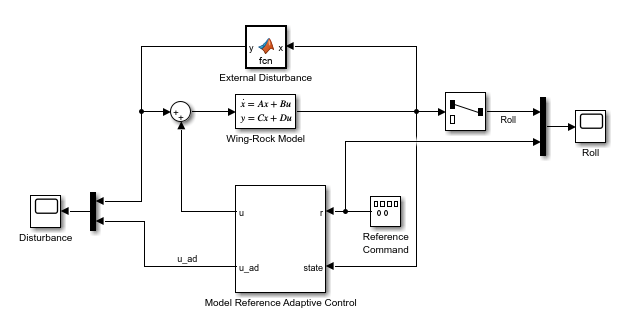In this model:

• The Wing-Rock Model block implements the nominal model of the roll dynamics.

• The External Disturbance block generates a wing-rock disturbance to the roll dynamics.

• The Reference Command block generates pilot reference signal.

• The Model Reference Adaptive Control block outputs the control action ${\mathit{u}}_{\mathrm{ad}}$, which is an estimate of the wing-rock disturbance.

The Model Reference Adaptive Control block is initially configured to use the states from the Wing-Rock Model block as the disturbance model features.

Set the simulation duration and simulate the model.

Tf = 40;    % Simulation duration (s)
sim(mdl);

Compare the resulting aircraft roll to the reference command.

open_system(mdl + "/Roll")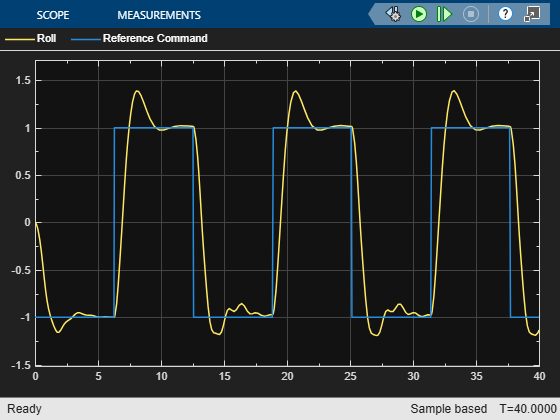The controller does not achieve a smooth second-order transient response.

Compare the disturbance model estimated by the MRAC controller with the true disturbance signal.

open_system(mdl + "/Disturbance")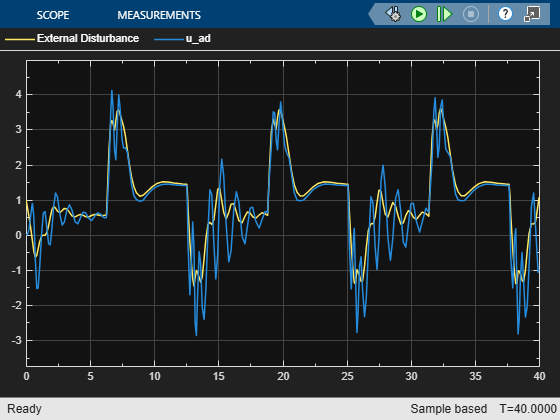When using the states as the disturbance model features, the controller does not accurately estimate the disturbance model.

Modify the MRAC controller to use 20 radial basis functions with centers spanning the range [-1 1]. Use the same bandwidth for all the basis function kernels.

controller = mdl + "/Model Reference Adaptive Control";
set_param(controller,'nCen','N')
set_param(controller,'cSpanMin','cen_min')
set_param(controller,'cSpanMax','cen_max')
set_param(controller,'cSig','bandwidth')

Simulate the model.

sim(mdl);

View the resulting controller performance.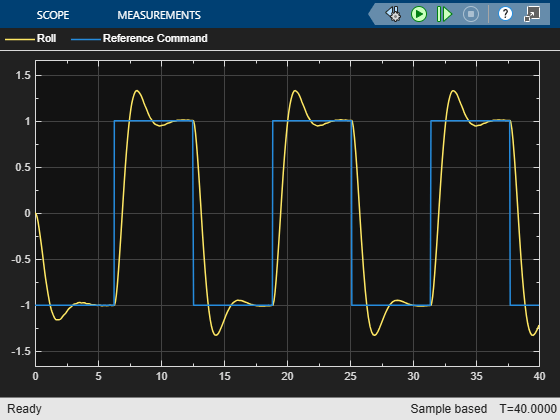The controller achieves a smoother second-order response for changes in the reference command.

Compare the disturbance estimated using the radial basis functions to the true disturbance.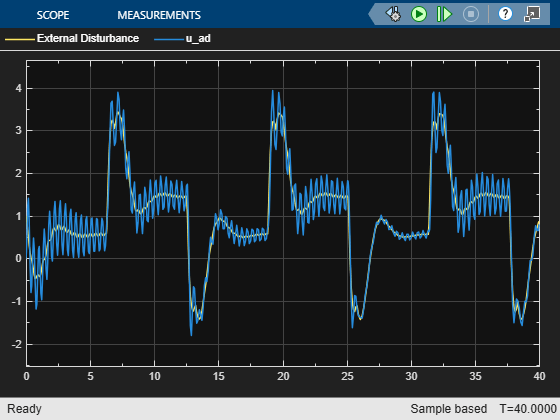As expected, using the radial basis functions as disturbance model features produces a more accurate model of the nonlinear disturbance.